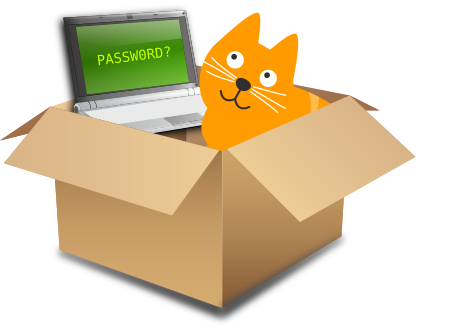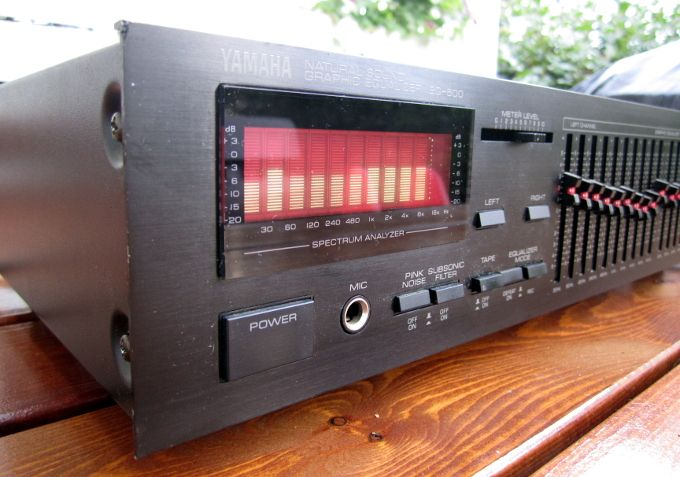Quantum computers have different properties than classical computers. This can be used to make some calculations dramatically faster, which in turn has implications for encryption. For example, RSA can be trivially broken given a quantum computer. This article explains approximately how this works.

## Cats in superposition

Some scientists proposed in 1935 that on a very small scale, particles can be in a superposition. Quantum computers use particles in superpositions to perform calculations. Bits in a classical computer can only be one or zero, but quantum bits can also be in a superposition: both one and zero at the same time. This can be used to perform computations that are not possible in the non-quantum, classical world.

Schrödinger made superposition more tangible by connecting the particle to a cat in a closed box. The particle and the cat are connected in some way, such that the cat is either alive or dead, depending on the state of the particle. In a classical world, when you open the box and you see a dead cat, you know that the cat has been dead all along. However, in a quantum world, the cat is in a superposition. It is both alive and dead at the same time until you open the box. When you open the box and look inside, only then the universe determines the fate of the cat.

This seems strange to most people. This is because quantum particles act like nothing in the classical world. In fact, quantum particles don’t act like cats in boxes. This also means that most of this article is factually incorrect, but it may still give you some feeling about how quantum calculations work.## Quantum speedup

Quantum computers work differently than classical computers, and can be used to perform some calculations much faster than classical computers. However, quantum computers are not faster at everything. Instead, quantum computers can perform different operations than classical computers. This makes it possible to use other algorithms with different timing characteristics. Quantum computers are particularly faster when they can take advantage of superposition to perform calculations on all possible inputs simultaneously. This is called quantum parallelism.

In this post, we are particularly interested on the effect on cryptosystems. That is because with crypto, an increase in speed means a reduction of security. Many cryptosystems are based on the fact that calculating something is hard or takes a long time. This may no longer hold for quantum computers.

Imagine we have a password protected laptop, and we want to crack the password. We could try every password until we get the correct one, but that would take a very long time. Instead, we may be able to use quantum to speed up the process.

We put the laptop along with the cat in the box. We instructed the cat beforehand to enter a random password. When we close the box, there is a superposition of cats that all try a different random password. In each parallel universe, the cat tries a different password. It is as if the cat in the box tries each password in parallel. However, as soon as we open the box, the state collapses and we end up with a classical situation. The cat tried only one password and it was probably the wrong one.

Now we do the same again, but we instruct the cat to enter a random password and climb out of the box if it is the correct one. We close the box, the cat is again in a superposition. However, this time the box opens and collapses to the state we want: the cat has entered the correct password. We obtained the password instantly, and in the classical world the password was just entered once. We used quantum parallelism to get lucky the first time.## Increasing correct cat probability

Actually, it doesn’t really work like that. We can’t tell the cat with the correct password to come out of the box. Remember, as soon as we look in the box the quantum state collapses and we end up with just one cat that tried one password. The test whether we have the correct password is similar to looking in the box. We can’t use if-statements when instructing the cats.

However, we can use quantum operations to increase the probability that the cat with the correct password comes out of the box when we open it. When the cat is in a superposition, we can perform operations that work on all cats. Some of these operations even change the probability that a certain cat will come out of the box as soon as we open it. We can increase the probability for cats whose outcome deviates from the average. In other words, the cat with the correct password is more likely to come out of the box. This process is called amplitude amplification. The price we pay for this is that the cat needs to enter multiple passwords, instead of just one.

Let’s say the laptop has an eight-digit password consisting of just letters. If we want to try them all, we have to type in 268 ≈ 2 × 1011 passwords. The cat in the box only needs to try the square root of that number, 456,976 passwords. This will still take a long time, but it is the difference between a week and 6,000 years of cracking. When we open the box, we will have almost a 100% chance of finding the cat with the correct password in it.

## Speed and limitations

Our cat algorithm provides a quadratic speedup compared to classical cracking. If we want to try N things in the classical world, we only have to do √N tries in the quantum computer. This cuts the “security bits” in half: a quantum computer can find a SHA512 hash (of 512 bits) as fast as a classical computer can reverse a SHA256 hash (of 256 bits). A common defense against this attack is thus to double the number of bits used in an algorithm.

We haven’t yet said anything about how the laptop checks the password. The cracking cat algorithm makes no assumptions about that. This means that it works for cracking any password check. As we’ll see later, we can crack some specific algorithms even faster. However, the password check we want to crack must be implemented as a quantum algorithm. If you want to reverse a MD5 hash, you first have to implement MD5 as a quantum algorithm. You can’t give the cat a classical laptop, it has to be a quantum laptop that can be in a superposition just like the cat.

## RSA

RSA is a public-private key cryptosystem. Something encrypted with the public key can only be decrypted with the private key, and vice versa. This works because of math. The exact math of encryption and decryption is not important right now. What we are interested in is the key generation algorithm:

• Pick two random big primes, p and q.
• Multiply these two primes to get the modulus of our keys: N = p × q
• Pick some small prime, e. This together with the modulus is the public key: (e, N)
• Calculate the private key (d) from the two random big primes (p, q) and the one small prime (e).

I won’t describe here how to calculate the private key. The point is that you can do so if you have the two random big primes and the small prime.

Assume that we have the public key of someone. That means we have the small prime e, and the modulus N. If we find which two primes p and q multiplied give the modulus N, we can calculate the private key, and we have broken RSA.

## Shor’s algorithm

That is what Shor’s algorithm does. Given a number, it calculates which two prime numbers can be multiplied to yield that number. For example, if you give it 15 it will return 3 and 5. This can obviously be used to break RSA, since we can break up the modulus from the public key into the two primes originally used to create the key. We can use these two primes to calculate the private key, which is supposed to be kept secret.

Shor’s algorithm uses quantum computing to find a period of a function, and then uses some more classical math to use that period to factor the modulus into two primes.

## Period finding

A Fourier transform splits a wave in separate frequencies. It is typically displayed on stereo amplifiers or audio players. It converts the wave into a histogram of which frequencies are present in the wave.

It is also possible to compute a Fourier transform over a function. This will show which “frequencies” are present in the function. In classical computers, we would first have to compute all outcomes of the function, and pass those to the Fourier transform. In quantum computers, however, we call the function once on a superposition. This in turn results in a superposition, which we can pass directly to the quantum Fourier transform.

If we ask the cat in the box to compute one value, we will have just one value when we open the box. But instead we take all parallel cats and compute some property (the frequency) on all of them. When we open the box, there will be one frequency that occurs a lot in the function.## Clock math

Clocks work modulo 12. Two hours after eleven o’clock is one o’clock, because 11 + 2 = 1 (mod 12). Now, let’s say we want to find the period of f(x) = 9x (mod 12). The result of this function is 0, 9, 6, 3, 0, 9, 6, 3, 0, etc. As you can see it repeats after four times. This is the period (or order) of this function: 4. Our previously mentioned quantum Fourier algorithm can find that fast.

The period always cleanly divides the modulus. Furthermore, if the period is even, then 6 is always halfway the period. The half of the modulus can be found at half of the period.

Suppose we have a very big clock of which we don’t know the size. We would very much like to know the size of the clock. If we find the period p of f(x) = 9x (mod ?) using our quantum Fourier function, we know that halfway the period we find the half of the size of the clock. So the size of the clock equals 2 × f(p).

## How this works for RSA

In RSA, the modulus is published as part of the public key. Unlike the clock example, this is not equal to the period. The modulus is made by multiplying two primes, p and q, together. The order of the function is equal to (p-1)(q-1). We use our clock math algorithm to found some period that evenly divides (p-1)(q-1). From this we can calculate p and q, and thus the private key.

## Some math

• We find the period r of the function f(x) = ax mod N. This loops us around the ring (“clock”) in r steps.
• This means that it loops around half the ring in ½r steps. ar/2 × ar/2 = ar = 1 mod N.
• Define z = ar/2. Replace ar/2 in the previous calculation by z: z × z = 1 mod N
• This is the same as z2 = 1 mod N
• Subtract 1 from each side: z2 - 1 = 0 mod N = N mod N
• This is the same as (z-1)(z+1) = 0 mod N = N mod N
• So the solutions are p = z-1 (mod N), q = z+1 (mod N)

## Conclusion

On a quantum computer we can simply calculate the private key from the public key for RSA.

No cats were hurt in the making of this blog post.